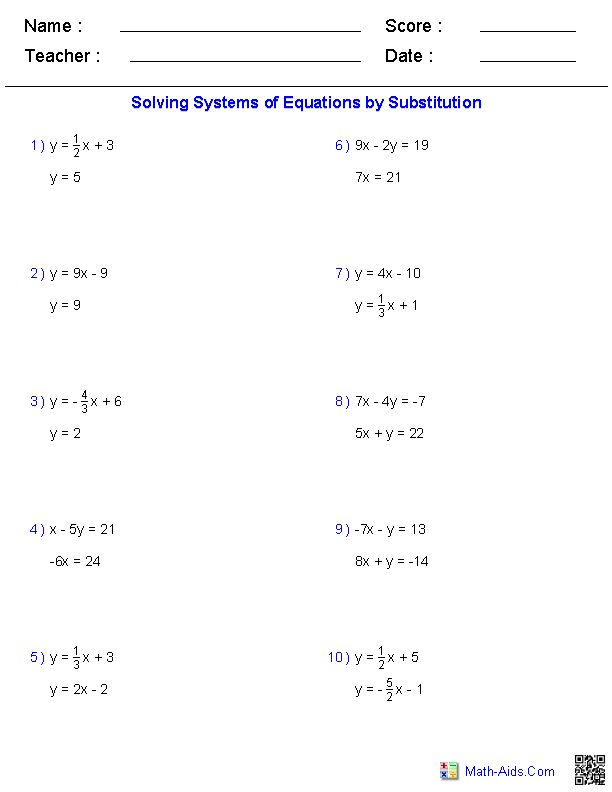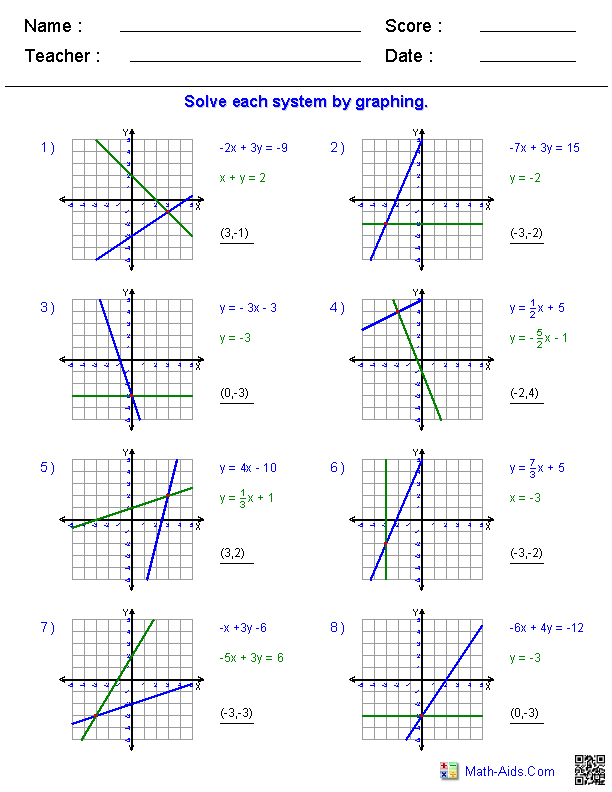#Pre-Algebra Worksheets

## Systems of Equations Worksheets

Here is a graphic preview for all of the Systems of Equations Worksheets. You can select different variables to customize these Systems of Equations Worksheets for your needs. The Systems of Equations Worksheets are randomly created and will never repeat so you have an endless supply of quality Systems of Equations Worksheets to use in the classroom or at home. Our Systems of Equations Worksheets are free to download, easy to use, and very flexible.

Click here for a Detailed Description of all the Systems of Equations Worksheets.

## Quick Link for All Systems of Equations Worksheets

Click the image to be taken to that Systems of Equations Worksheets.

##### Handout forSystems of EquationsWorksheets##### Solving AlgebraicallyTwo VariableSystems of EquationsWorksheets##### Solving GraphicallyTwo VariableSystems of EquationsWorksheetsRecommended Videos

## Description For All Systems of Equations Worksheets

Handout for Systems of Equations Worksheets
This systems of equations worksheet will produce a seven page handout for the students concerning Systems of Equations. You may select which methods will be produced for the handout. This monomials worksheet is a good resource for students in the 5th Grade, 6th Grade, 7th Grade, and 8th Grade.

Solving Algebraically Two Variable Systems of Equations Worksheets
This systems of equations worksheet will produce problems for solving two variable systems of equations algebraically. You may select which type of method the student should use to solve the problems. This systems of equations worksheet will produce ten problems per page. This systems of equations worksheet is a good resource for students in the 5th Grade, 6th Grade, 7th Grade, and 8th Grade.

Solving Graphically Two Variable Systems of Equations Worksheets
This systems of equations worksheet will produce problems for solving two variable systems of equations graphically. You may select four different types of problems. This systems of equations worksheet will produce eight problems per page. This systems of equations worksheet is a good resource for students in the 5th Grade, 6th Grade, 7th Grade, and 8th Grade.